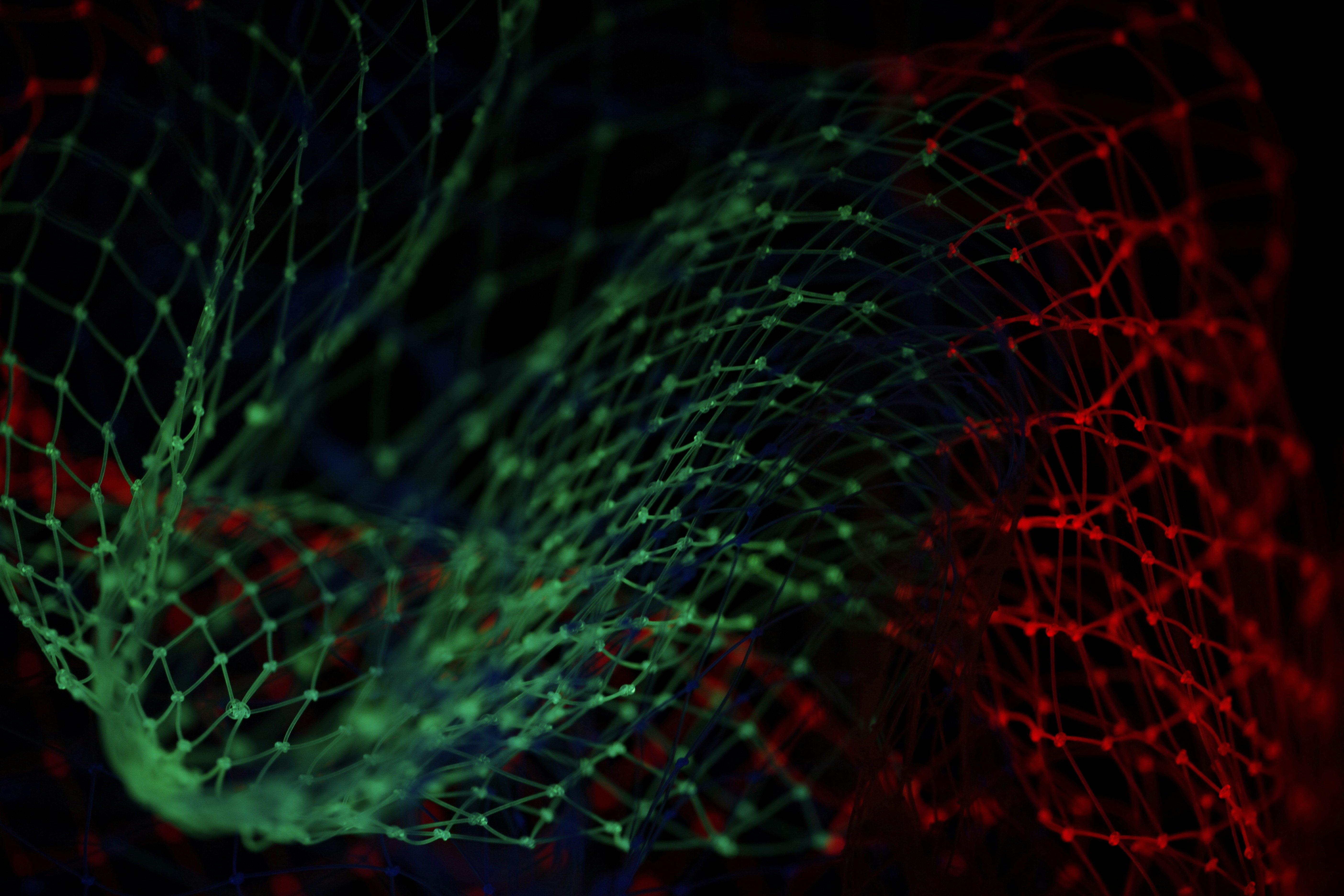# Mohamed Elmahdy

Director of Data Science at Raisa## Inductive Clustering For Lower Dimension Input Vectors

IntroductionWe often need to expand or generalize the output of clustering algorithms for unseen data points in training without recomputing the clustering. Some clustering algorithms support this by nature, which is called Inductive Clustering. This article will discuss extending the non-inductive clustering algorithms to classify new instances and classify data## Time Series Forecasting using Transformers and Ordinal Regression

Raisa's Domain and Time Series PredictionOne of the things we do at Raisa is forecasting oil and gas production for all wells in the United States. Forecasting are usually done for the next twelve months based on previous data, which is by definition a time series forecasting problem.With the## Introduction to Probabilistic Deep Learning

Why Bayesian deep learning?Have you ever tried to learn about Bayesian neural networks but got hit in the face with a barrage of equations? Have you ever implemented a Bayesian neural network without actually understanding why this works? If the answer is yes to any of those questions, then